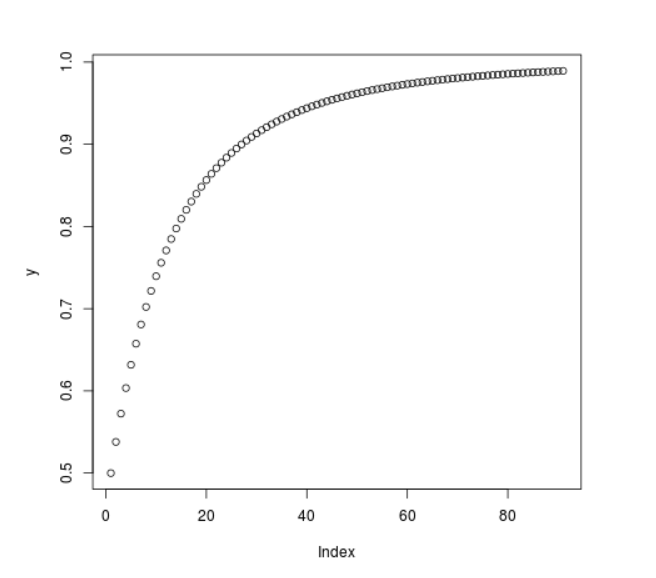# Compute Cumulative Log Normal Probability Density in R Programming – plnorm() Function

`plnorm()` function in R Language is used to compute the log normal value of the cumulative probability density function. It also creates a plot of the cumulative distribution of log normal density.

Syntax: plnorm(vec)

Parameters:
vec: x-values for normal density

Example 1:

 `# R program to compute cumulative ` `# log normal probability density ` ` `  `# Creating x-values for density ` `x <``-` `seq(``1``, ``10``, by ``=` `1``) ` ` `  `# Calling plnorm() function ` `y <``-` `plnorm(x) ` `y `

Output:

```  0.5000000 0.7558914 0.8640314 0.9171715 0.9462397 0.9634142 0.9741672
 0.9812116 0.9859978 0.9893489
```

Example 2:

 `# R program to compute cumulative ` `# log normal probability density ` ` `  `# Creating x-values for density ` `x <``-` `seq(``1``, ``10``, by ``=` `0.1``) ` ` `  `# Calling plnorm() function ` `y <``-` `plnorm(x) ` ` `  `# Plot a graph ` `plot(y) `

Output:My Personal Notes arrow_drop_upCheck out this Author's contributed articles.

If you like GeeksforGeeks and would like to contribute, you can also write an article using contribute.geeksforgeeks.org or mail your article to contribute@geeksforgeeks.org. See your article appearing on the GeeksforGeeks main page and help other Geeks.

Please Improve this article if you find anything incorrect by clicking on the "Improve Article" button below.

Article Tags :

Be the First to upvote.

Please write to us at contribute@geeksforgeeks.org to report any issue with the above content.